# Natural sequence

(diff) ← Older revision | Latest revision (diff) | Newer revision → (diff)

natural number sequence

The non-empty setin which a unary operationis defined (i.e.is a single-valued mapping of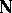into itself) satisfying the following conditions (the Peano axioms):

1) for any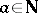,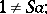2) for any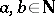: If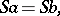then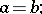3) any subset ofthat contains 1 and that together with any elementalso contains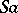, is necessarily the whole of(axiom of induction).

The elementis usually called the immediate successor of. The natural sequence is a totally ordered set. It can be proved that the conditions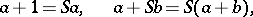whereandare arbitrary elements of, define binary operationsandon. The system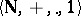is the system of natural numbers (cf. Natural number).

How to Cite This Entry:
Natural sequence. Encyclopedia of Mathematics. URL: http://encyclopediaofmath.org/index.php?title=Natural_sequence&oldid=16451
This article was adapted from an original article by A.A. BukhshtabV.I. Nechaev (originator), which appeared in Encyclopedia of Mathematics - ISBN 1402006098. See original article